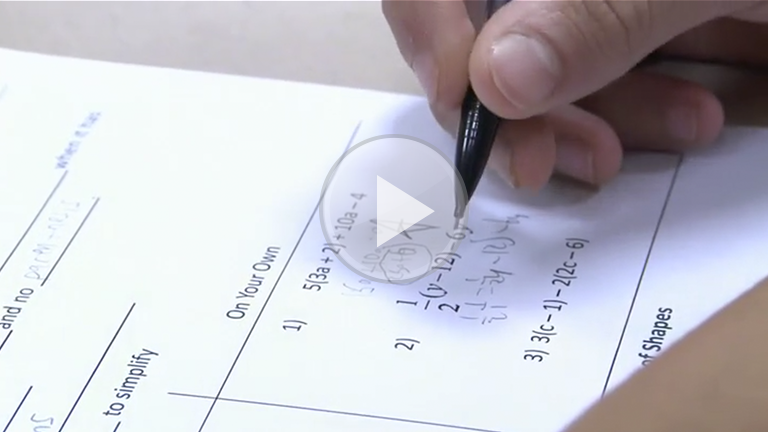TAGS: Grade Level Middle (5 – 8) High (9 – 12) Subject Math Topic/Practice Evidence of Learning Clear Learning Targets Date 12/22/2014 Turning Guided Notes into a Formative ToolDESCRIPTION Learn how to use guided notes in your classroom and use them as a tool for formative assessment.I can add, subtract, factor and expand linear expressions with rational coefficients in order to identify and write equivalent expressions. I can rewrite an expression in different forms to show how the quantities are related. I can combine like terms and use the distributive property to simplify expressions. I can identify, analyze and write equivalent expressions.CCSS.MATH.CONTENT.7.EE.A.1 Apply properties of operations as strategies to add, subtract, factor, and expand linear expressions with rational coefficients. CCSS.MATH.CONTENT.7.EE.A.2 Understand that rewriting an expression in different forms in a problem context can shed light on the problem and how the quantities in it are related. For example, a + 0.05a = 1.05a means that "increase by 5%" is the same as "multiply by 1.05." CONFIRM YOUR LEARNING How do Mrs. Graf’s students benefit from the guided notes that have both math problems that teacher and students do together and problems that students do on their own? How does Mrs. Graf use guided notes as formative assessment? How do the guided notes used in Mrs. Graf’s class help students take ownership of their learning? CONFIRM YOUR PRACTICE How can you use guided notes with your students? How can guided notes benefit your students? CONFIRM YOUR COMMITMENT What process will you try to help your students organize their learning? How can the process that you choose double as a formative assessment?